Science, Maths & Technology

### Become an OU student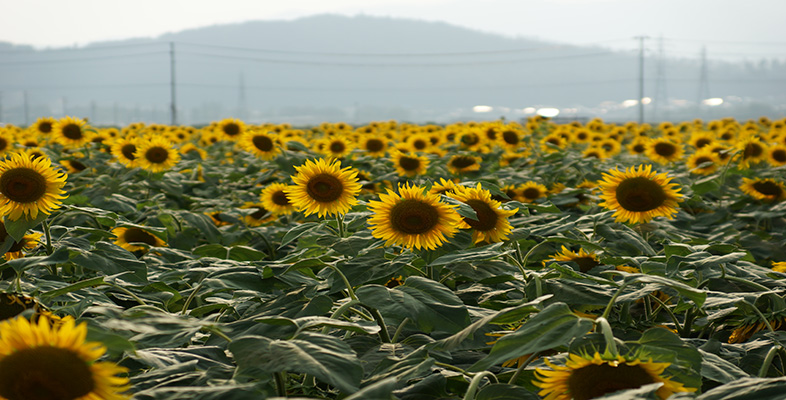Ratio, proportion and percentages

Start this free course now. Just create an account and sign in. Enrol and complete the course for a free statement of participation or digital badge if available.

# 3.2 Converting to a percentage

Fractions and decimals can also be converted to percentages, by multiplying by 100%.

So, for example, 0.17, 0.3 andcan be expressed as percentages as follows:

0.17 × 100% = 17%;

0.3 × 100% = 30%;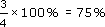Decimals or fractions bigger than 1 correspond to percentages greater than 100%. For example,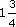= 1.75, which as a percentage is 1.75 × 100% = 175%.

Care needs to be taken when talking in percentages. A percentage is a percentage of a given quantity: 50% of the voters, 25% of the budget, 10% of the population. In newspapers, it is not always clear what quoted percentages are percentages of. Politicians too can give misleading statements. ‘We are giving an increase in funding of 10% and 5% of this has no strings attached’. In this statement it is not clear whether the 5% is 5% of the original funding or 5% of the 10% increase in funding (which would be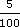× 10% = 0.5% of the original funding).

To avoid this confusion some people use the term percentage points, when they are comparing the percentages of different quantities. In elections the percentage of the vote at one election for a given party is often compared with the percentage of the vote at the previous election. The difference is referred to as the ‘swing’ and expressed in percentage points.

## Example 11

In a local election 2540 votes are cast for the Purple party out of a total of 5000 votes. At the previous election 42.1% of votes had been for the Purple party. What is the swing to the Purple party in percentage points?

2540 out of 5000 as a percentage is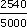× 100 = 50.8%